Home > GC > Chapter 8 > Lesson 8.2.2 > Problem8-76

8-76.
1.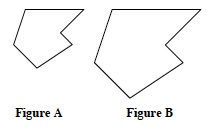Assume Figure A and Figure B, at right, are similar. Homework Help ✎

1. If the ratio of similarity is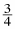then what is the ratio of the perimeters of A and B?

2. If the perimeter of Figure A is p and the linear scale factor is r, what is the perimeter of Figure B?

3. If the area of Figure A is a and the linear scale factor is r, what is the area of Figure B?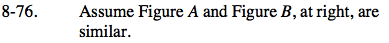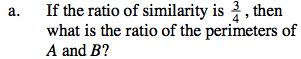$\frac{3}{4}$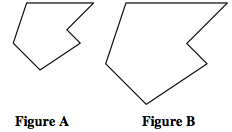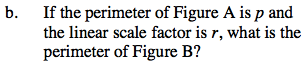Use the ratio of similarity to find the perimeter.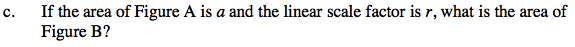If the linear scale factor is r, what is the area ratio?